Tuesday, June 22, 2021
Home > Latest Announcement > NMTC 2017 Question Papers With Solutions Sub Junior Level

# NMTC 2017 Question Papers With Solutions Sub Junior Level# NMTC 2017 Question Papers with Solutions Sub Junior Level

NMTC is an acronym for National Mathematics Talent Contest. NMTC is conducted by AMTI (Association of Mathematics Teachers of India) to identify the talent of the students in Mathematics. In this post, NMTC 2017 Question Papers with Solutions Sub Junior Level is published.

Part A: Instruction:

Only One of the choices A, B, C, D is correct. For each correct response, you get 1 mark. For each incorrect response, you lose 1/2 mark.

NMTC 2017 Paper For Sub Junior Level Ques No 1:

The fraction 4/37 is written in the decimal form 0.a1a2a3….. The value of a2017 is

Options:

A. 8

B. 0

C. 1

D. 5

Solution:

4/37 = 0.108108108…

2017 is divisible by 3. Thus, the remainder is 1. Therefore, a2017 digit is 1.

NMTC 2017 Paper For Sub Junior Level Ques No 2:

The number of integers x satisfying the equation (x2 – 3x + 1)x + 1 = 1 is

Options:

A. 2

B. 3

C. 4

D. 5

Solution:NMTC 2017 Paper For Sub Junior Level Ques No 3:

The number of two digit numbers ab such that the number ab – ba is a prime number is

Options:

A. 0

B. 1

C. 2

D. 3

Solution:

ab – ba = 10a + b – 10b – a = 9a – 9b = 9(a – b). So, 9 is always the factor of ab – ba. So, there is no such number is present.

NMTC 2017 Paper For Sub Junior Level Ques No 4:

If A = 5425/1444 + 2987/3045 – 493/4284

Options:

A. 1 < A < 2

B. 2 < A < 3

C. 3 < A < 4

D. A <1

Solution: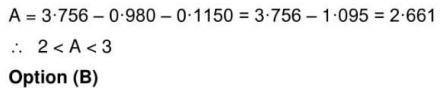NMTC 2017 Paper For Sub Junior Level Ques No 5:

What is the 2017th letter in ABRACADABRAABRACADABRA…, where the word ABRACADABRA is repeatedly written?

Options:

A. A

B. B

C. C

D. R

Solution: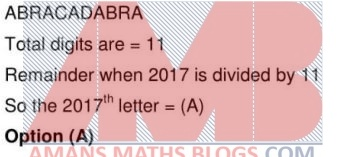NMTC 2017 Paper For Sub Junior Level Ques No 6:

How many of the following statements are true?

1. A 10% increase followed by another 5% increase is equivalent to a 15% increase.

2. If the radius of a circle is doubled then the ratio of the area of the circle to the circumference is doubled.

3. If a positive fraction is subtracted from 1 and the resulting fraction is again subtracted from 1 we get the original fraction.

Options:

A. 0

B. 1

C. 2

D. 3

Solution: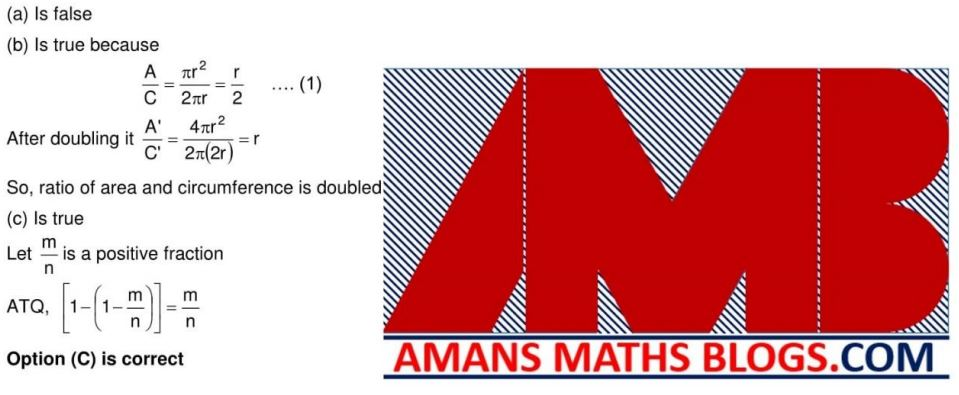NMTC 2017 Paper For Sub Junior Level Ques No 7:

In the adjoining figure the breadth of the rectangle is 10 units. Two semicircles are drawn on the breadth as diameter. The area of the shaded region is 100 sq units. The shortest distance between the semicircles isOptions:

A. 5Pi/2

B. 5Pi

C. 5Pi/3

D. 3Pi/4

Solution: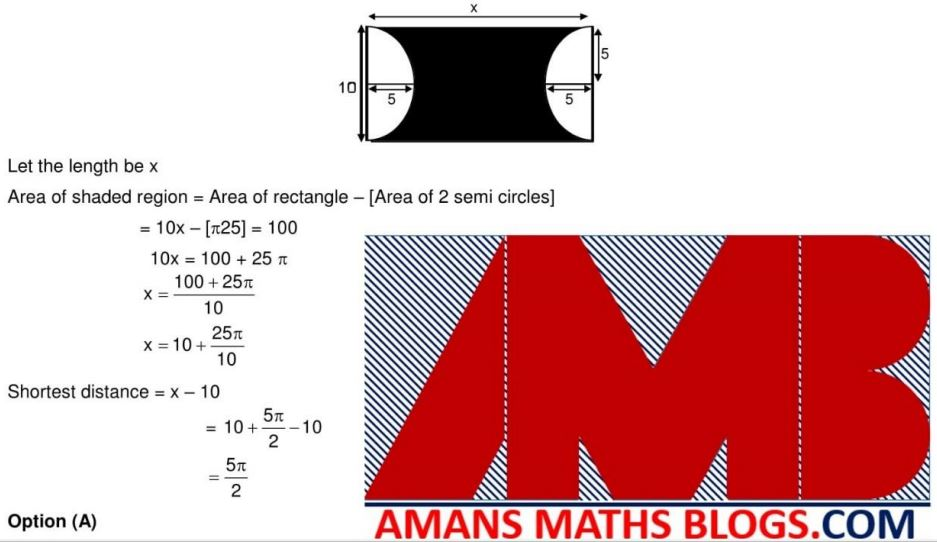NMTC 2017 Paper For Sub Junior Level Ques No 8

When you arrange the following in descending order: (A) 15% of 30, (B) 8% of 15, (C) 20% of 20, (D) 26% of 10, (E) 9% of 25. The middle one is

Options:

A. 15% of 30

B. 8% of 15

C. 20% of 20

D. 26% of 10

Solution: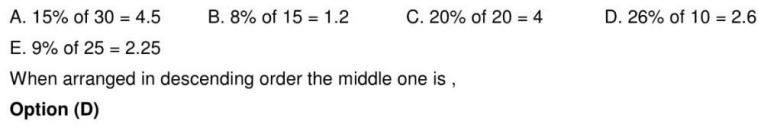NMTC 2017 Paper For Sub Junior Level Ques No 9

A boy aims at a target shown in the figure. When he hits the center circle he gets 7 points, first annular region 3 points. He shoots six times. Which one of the following is a possible score?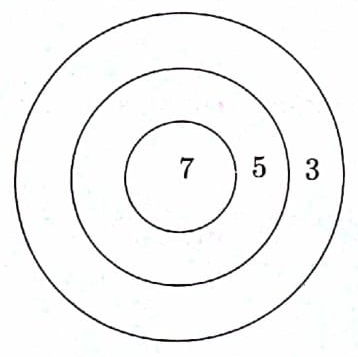Options:

A. 16

B. 26

C. 19

D. 41

Solution:NMTC 2017 Paper For Sub Junior Level Ques No 10

After simplifying the fraction, We get a term independent of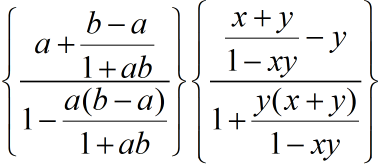Options:

A. a, y

B. b, x

C. a, b

D. x, y

Solution: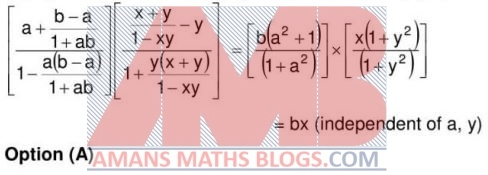NMTC 2017 Paper For Sub Junior Level Ques No 11

If 7 Rasagullas are distributed to each boy of group, 10 Rasagullas would be left. If 8 are given to each boy then 5 Rasagullas would be left. So the person who distributes the Rasagullas brought 15 more Rasagullas and distributed the same number (x) Rasagullas to each. There is no Rasagullas left. Then x is

Options:

A. 10

B. 11

C. 12

D. 14

Solution: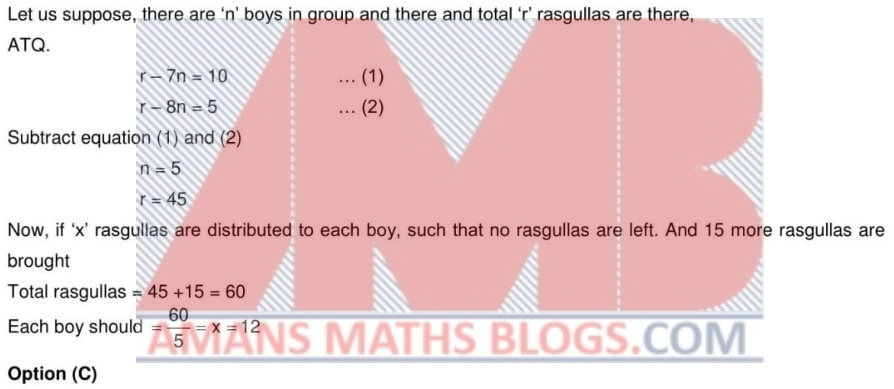NMTC 2017 Paper For Sub Junior Level Ques No 12

In the adjoining diagram all square are of the same size. The total area of the figure is 288 square cms. The perimeter of the figure (in cm) is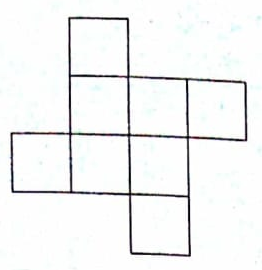Options:

A. 86

B. 96

C. 106

D. 92

Solution: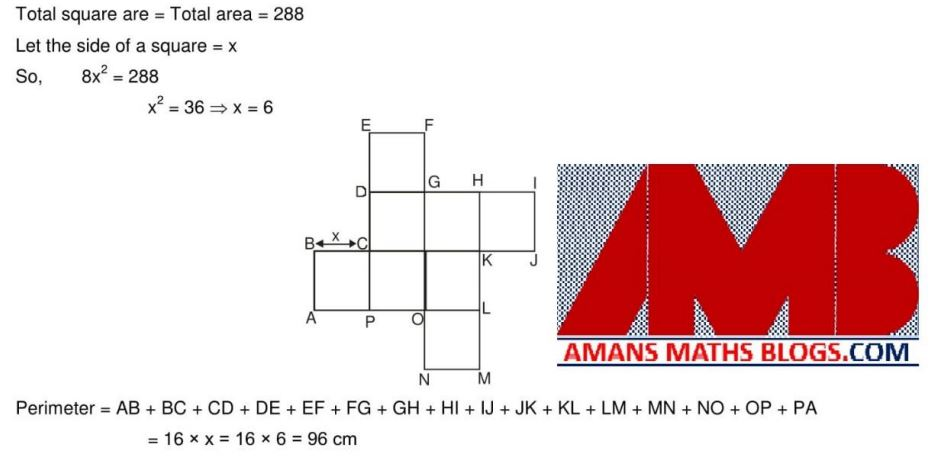NMTC 2017 Paper For Sub Junior Level Ques No 13

When Newton was a primary school student he had to multiply a number by 5. But by mistake he divided the number by 5. The percentage error he committed is

Options:

A. 95%

B. 96%

C. 50%

D. 75%

Solution: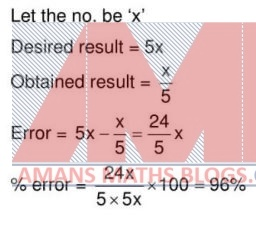NMTC 2017 Paper For Sub Junior Level Ques No 14

ABC is an isosceles triangle with sides AB = AC = 3x – 4 = 3x/4 + 32. The area of the equilateral triangle with side length x is

Options:

A. 32B. 36C. 54D. 64Solution: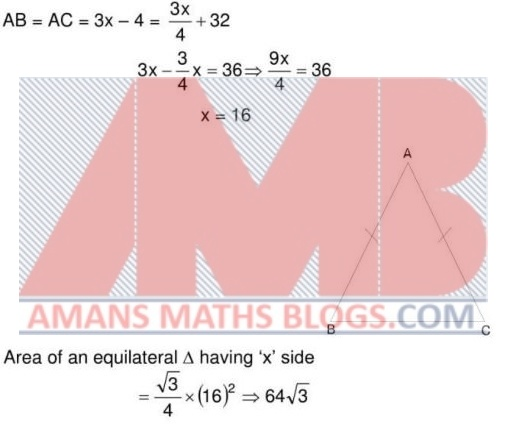NMTC 2017 Paper For Sub Junior Level Ques No 15

Two distinct numbers a and b are selected from 1, 2, 3, . . . 60. The maximum value of ab/(a – b) is

Options:

A. 6750

B. 5270

C. 4850

D. 3540

Solution:PART B: NMTC 2017 Question Papers with Solutions Sub Junior Level

NMTC 2017 Paper For Sub Junior Level Ques No 16

Two cogged wheels of which one has 16 cogs and the other 27 cogs, mesh into each other. If the latter turns 80 times in three quarters of a minute, the number of turns made by the other in 8 seconds is_______

Solution: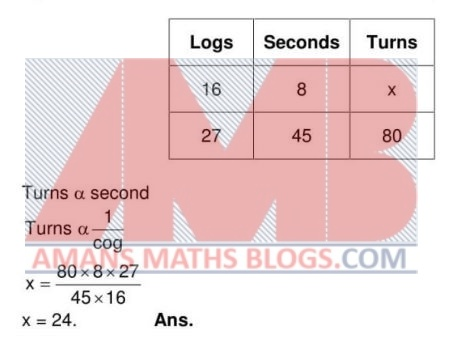NMTC 2017 Paper For Sub Junior Level Ques No 17

If n is a positive integer such that a2n = 2, then 2a6n – 16 is_______.

Solution:NMTC 2017 Paper For Sub Junior Level Ques No 18

The least number of children in a family such that every child has at least one sister and one brother is________.

Solution:

Family should have at least four children (2 male and 2 female).

NMTC 2017 Paper For Sub Junior Level Ques No 19

A water tank is 4/5 full. When 40 liters of water is removed, it becomes 3/4 full. The capacity of the tank in liters is_______.

Solution:NMTC 2017 Paper For Sub Junior Level Ques No 20

ABC is an equilateral triangle. Squares are describe on the sides AB and AC as shown. The value of x is______.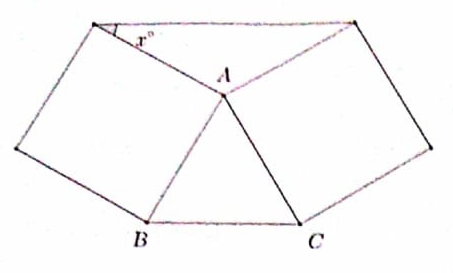Solution:NMTC 2017 Paper For Sub Junior Level Ques No 21

ABCD is a trapezium with AB = 6 cm, AD = 8 cm and CD = 18 cm. The sides AB and CD are parallel and AD is perpendicular to AB. P is the point of intersection of AC and BD. The difference between the areas of the triangle PCD and PAB is ______.Solution:NMTC 2017 Paper For Sub Junior Level Ques No 22

The price of cooking oil has increased by 25%. The percentage of reduction that a family should effect in the use of oil so as not to increase the expenditure is _______.

Solution: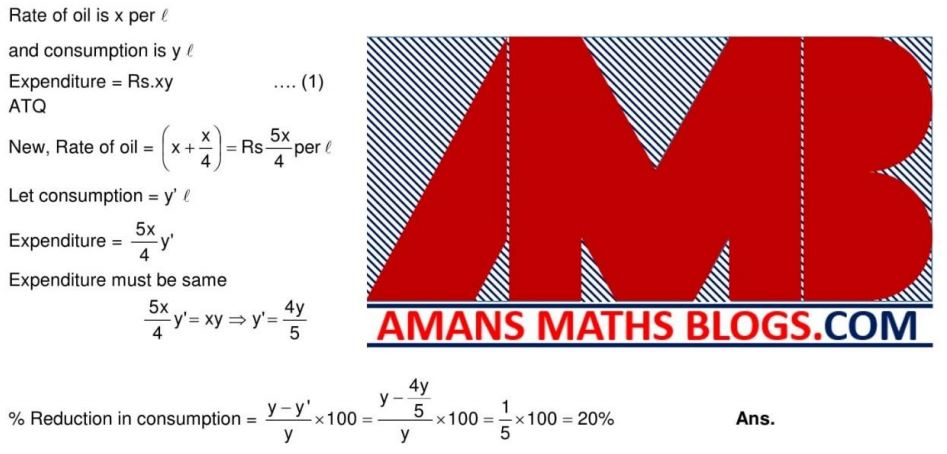NMTC 2017 Paper For Sub Junior Level Ques No 23

The number of natural numbers between 99 and 999 which contains exactly one zero is ____.

Solution: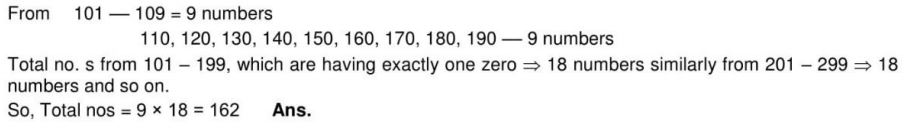NMTC 2017 Paper For Sub Junior Level Ques No 24

In the adjoining figure, we have semicircle and AB = BC = CD. The ratio of the unshaded area to the shaded area is _____.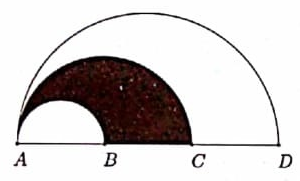Solution:NMTC 2017 Paper For Sub Junior Level Ques No 25

Gold is 19 times as heavy as water and copper is 9 times as heavy as water. The ratio in which these two metals be mixed so that the mixture is 15 times as heavy as water is ____.

Solution:NMTC 2017 Paper For Sub Junior Level Ques No 26

Five angles of a heptagon (seven sides polygon) are 160 degree 135 degree, 185 degree, 145 degree and 125 degree. If the other two angles are both equal to x degree, then x is _____.

Solution: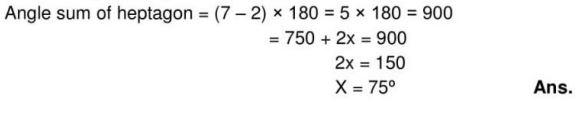NMTC 2017 Paper For Sub Junior Level Ques No 27

ABCD is a trapezium with AB parallel to CD and AD perpendicular to AB. If AB = 23 cm. CD = 35 cm and AD = 5 cm. The perimeter of the given trapezium in cm is _____.

Solution: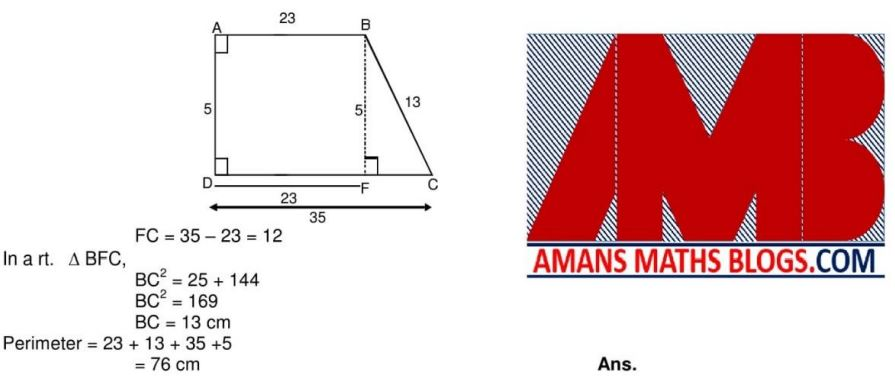NMTC 2017 Paper For Sub Junior Level Ques No 28

The number of three digit numbers which  are multiple of 11 is ____.

Solution:NMTC 2017 Paper For Sub Junior Level Ques No 29

If a, b are the digits, ab denotes the number 10a + b. Similarly, when a, b, c are digits, abc denotes the number 100a + 10b + c. If X, Y, Z are digits such that XX + YY + ZZ = XYZ, then (XX)x(YY)x(ZZ) is _____.

Solution: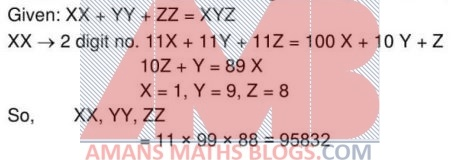NMTC 2017 Paper For Sub Junior Level Ques No 30

The positive integer n has 2, 5 and 6 as its factor and the positive integer m has 4, 8, 12 as its factors. The smallest value of m + n is ____.

Solution:error: Content is protected !!